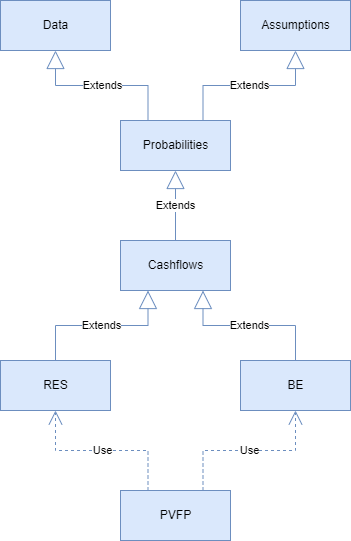# Object-oriented actuarial model

Jan 23, 2022

What’s the benefit of making an actuarial model object-oriented? Kaustav Sen developed an object-oriented actuarial model from scratch using modelx. The model calculates the present value of future profits and it’s a perfect example to demonstrate the benefit.

The structure of the model looks like below.Each rectangle in the diagram represents a Space, in which a set of formulas and data are defined.

To project future profits, you need to model reserves. Reserving methods vary by country-specific regulations and accounting purposes, but in many cases including this example, reserves and best-estimate cashflows are calculated by the same set of formulas, but with different assumption sets.

Modeling the same set of formulas with the different sets assumptions in this model is achieved by an object-oriented approach. In the base space named Cashflows, formulas that calculate cashflows are defined. The formulas are common between the best-estimate and reserve calculations. Then there are BE and RES spaces that inherit from Cashflows. Both BE and RES copy the same formulas from Cashflows, but they read different sets of assumptions from DataFrames inherited from the Assumption space.

Finally, another space named PV refers to BE and RES, to use the cashflows from BE and per-policy reserves from RES to calculated PVFP.

Now you see the benefit. Neither you need to define the same set of formulas twice for the best-estimate and reserving nor define a loop to iterate over the same set of formulas with different sets of assumptions, while saving the calculated values in variables separately from the formulas.